## General Mechanical

•Adisa
Subscriber

Hi everyone,

I need to do analysis, where I need to determine stress  will crack occur. I need to simulate the passage of the axle (red) through hole (black).  The diameter of the axle  is bigger then hole, how to do this. The axle is of steel and part which has hole is of plastic.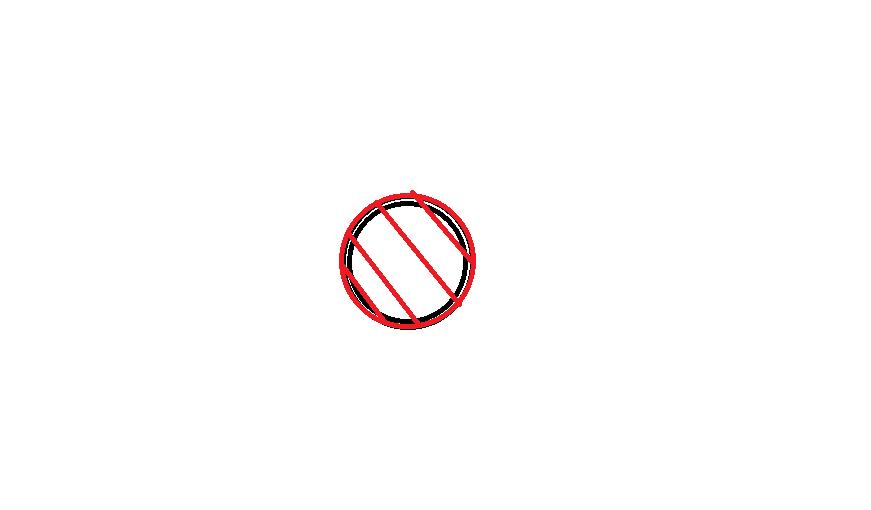The attached material of part with hole, and the criteria of crack, how to determine.

Best regards.

•Adisa
Subscriber

I get some results, but I am not sure what it means. Whether will  the crack occur on the part with hole, which the properties of material is attached on the first post for temperature 23. I need to determine phenomenon of crack based on the datasheet of material.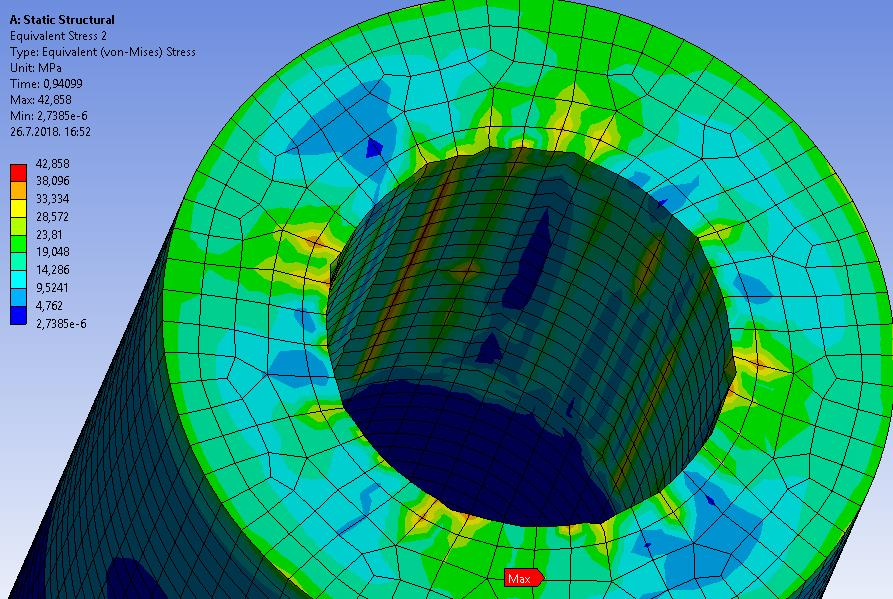and plastic strain is 0,186 it is 18%

Best regards.

•Adisa
Subscriber

Can anyone halp me?

•peteroznewman
Subscriber

You have a pin that is larger than the hole. There are two simulations that are of interest. The simplest one is the state of stress when the pin is fully inserted in the hole.  The complex simulation is the isertion of the tapered pin into the hole.  Let's do the simplest one first.

You have axisymmetric geometry and loading, which means you can do a 2D model, which will take less time to solve and will compute the stress in the plastic (and steel). I see you have a mulilinear plasticity model for the plastic.

The geometry is drawn of the two parts assembled and there is an interference present. The contact elements will resolve that interference. You don't need to move any parts, just support the bottom of the pin with a Y=0 displacement constraint. The axis of the pin will be on the Y axis.

The material datasheet shows the strain at break to be 4.5%. This means that in your simulation, when the Total Strain is > 4.5% the part cracked.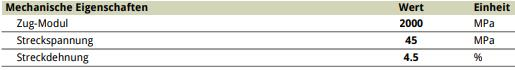You can use 1/2 symmetry and cut the pin and sleeve to half their length.  This is what the sketch will look like: two rectangular surfaces that overlap by the radial interference. The dimension H5 is half the internal diameter of the plastic. The dimension H2 is half the diameter of the pin.  Dimension V7 is half the length of the tube. When you create the second rectangle, Add Frozen. And before you start, change the Geometry property to 2D.  I didn't use your geometry, I made up my own, but the solver finished in seconds and made a result with your material. This my geometry, the prediction is that the plastic will not crack since total strain is 0.033 < 0.045.

Regards,

Peter

•Adisa
Subscriber

Thank you peter,

I did first simulation, can you help me to describe results and get me help for another analysis.

ansys18.2

For hex elements.

The red curve is results of stress and blue is input stress for multilinear isotropic hardening. The contact properties.

The below pic is for triangle elements.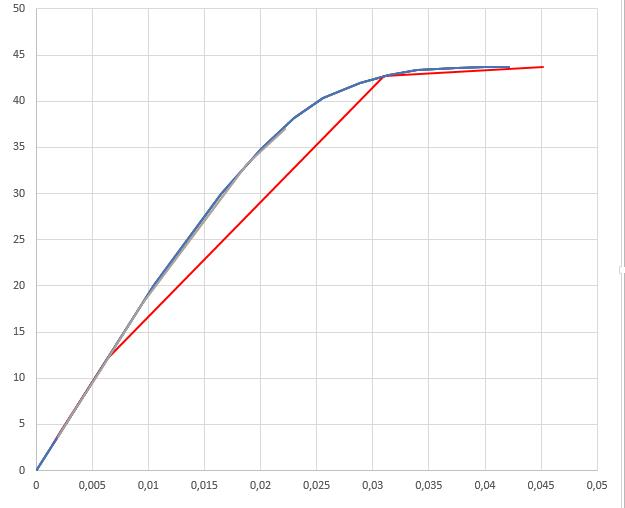I do with one shell another solid body. I have some errors  (DEVIATIONS from real stress) and i need to increase interations and refinement mesh, and if I have total strain bigger than 4,5 % the construction will cracked. OK.

Now I need to do second simulation, the complex simulation is the insertion of the tapered pin into the hole. I can also do the second analysis as the first, I need to determine stress on plastic part.

•Adisa
Subscriber

I did slice of the second analysis, and I get this results.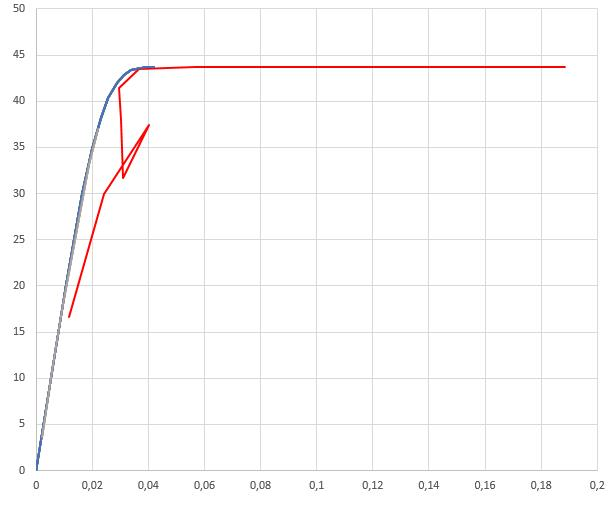Blue is input stress-strain, red is result.

The slice analysis is attached, one is solid another part is shell.

These analysis are examples for axle press, axle has teeth, where I need to apply to complex structure, where I can not use shell because construction is complex, and because it  I need this example do with solid.

In the complex with axle-teeth, The results of stress-strain look like this:

Tetr elements are used, solver is converged but the results have more error, devitation of real stress strain curve, what Ineed to do that cut down errors. the strain of 4,5 is exceed but the stress at 4,5% straiina is not 45MPa.

All  the best Peter, and thank you in advance.

•peteroznewman
Subscriber

First simulation

Why are you using shell elements on a surface instead of solid elements in a solid body?  There are special commands used to put more integration points through the thickness of a shell element. The default number might not give you the most accurate results. With solid elements you see how many elements are through the thickness of the plastic.

Displacement holds axial motion to zero at both ends. This is wrong, it should only be at one end, the cut plane of symmetry. The other end of the tube is free.

The red line on your chart seems to be a long way from the blue line, but the line is not data. Only the points at the end of each red line are data. Don't draw lines between the data points.

To make more points on the Charts, change the Analysis Setting of Minimum Substeps to 10.

Why plot a chart?  Why not plot what I did, which shows the distribution of strain through the thickness?

I will comment on the second analysis in the next post.

Regards,

Peter

•peteroznewman
Subscriber

Second analysis

Shell elements are not appropriate for this analysis.

Put two planes through the axis of the pin, lined up with the valley on each side of a tooth and slice out one tooth then use symmetry. You can then analyze one tooth with a detailed solid model. You will continue to analyze only one half of the length of the tube, so there will be a symmetry plane at the center of the tube length.

The tip of the tooth is not sharp, but has a very small radius on it. This tip radius may be required and small elements in the sleeve that can wrap around this radius may help get a detailed result.

Regards,

Peter

•Adisa
Subscriber

Peter thank you for all,

I plot stress strain curve, because I thought that real curve must be equal with results and that criteria must be and stress and strain.

I used stress and strain for crack, for example, the part will crack when is t.strain  bigger than 4,5 % and stress in that point must be 40 MPa, and that I used as verification analysis when is curve equal of results as input.

Can I use only total strain for criteria, for exmple that total strain is bigger than 4,5 % but stress do not equal 40MPa,  the result of stress is different of 40MPa. i.e Total strain is criteria for creck reckless on value of stress.

•peteroznewman
Subscriber

You should use Total Strain to compare with 4.5% to evaluate if the part would have cracked, regardless of stress.

With the shell model, the integration point can be far from the node. When you switch to small solid elements, the integration point will be close to the node. Plasticity is enabled on the element when the stress at the integration point passes a yield threshold in the material model.

When there is a large distance between the integration point and the node, the stress at the integration point may not have reached the yield threshold, but the stress extrapolated out to the nodes can be higher than the yield point. This error will be minimized when you have small solid elements.

Regards,

Peter

•Adisa
Subscriber

Thank Peter. I did the analysis following your the tips.

•Adisa
Subscriber

Peter,

If I use the axle  as rigid, Will it be wrong? Because the axle is of steel but another part is of plastic.

•Sandeep Medikonda
Ansys Employee

Adisa, If you don't expect it to deform much in comparison and if there is a big difference in the stiffness of 2 bodies, it should be ok.

Does your simulation take a long time to complete? I would typically run 2 cases, one with a rigid part and one with a steel body and compare when I am having a doubt like this.

•Adisa
Subscriber

Sandeep,

Thanks, I did two 2 cases, with rigid and flexible and the both results are similar, do not have much difference, and I can use rigid.

From this analysis I guess that the contact put on part with lower stiffness while the target on bigger. Remodel me If I am wrong.

All the best.

•peteroznewman
Subscriber

ANSYS requires you to put the Target on the Rigid part. It won't let you put it the opposite way.

Regards

•Sandeep Medikonda
Ansys Employee